##### Math For Real Life For DummiesA ratio is a mathematical comparison of two numbers, based on division. For example, suppose you bring 3 shirts and 5 ties with you on a business trip. Here are a few ways to express the ratio of shirts to ties:

3:5 3 to 5 3/5

A good way to work with a ratio is to turn it into a fraction. Be sure to keep the order the same: The first number goes on top of the fraction, and the second number goes on the bottom.

You can use a ratio to solve problems by setting up a proportion equation — that is, an equation involving two ratios.

## Sample questions

1. Clarence has 1 daughter and 4 sons. Set up a proportion equation based on this ratio.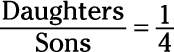2. An English language school has 3:7 ratio of European to Asian students. If the school has 28 students from Asia, how many students in the school are from Europe?

12 students. To begin, set up a proportion based on the ratio of European to Asian students (be sure that the order of the two numbers is the same as the two attributes that they stand for — 3 Europeans and 7 Asians):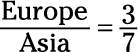Now, increase the terms of the fraction 3/7 so that the number representing the count of Asian students becomes 28: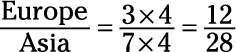Therefore, given that the school has 28 Asian students, it has 12 European students.

## Practice questions

1. A farmers market sells a 4 to 5 ratio of vegetables to fruit. If it sells 35 different types of fruits, how many different types of vegetables does it sell?

2. An art gallery is currently featuring an exhibition with a 2:7 ratio of sculpture to paintings. If the exhibition includes 18 sculptures, how many paintings does it include?

3. A summer camp has a 7 to 9 ratio of girls to boys. If it has 117 boys, what is the total number of children attending the summer camp?

4. The budget of a small town has a 3:8 ratio of state funding to municipal funding. If the town received \$600,000 from the state last year, what was its total budget from both state and municipal sources?

Following are the answers to the practice questions:

1. 28. To begin, set up a proportion based on the ratio of vegetables to fruit: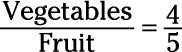Now, increase the terms of the fraction 4/5 so that the number representing fruit becomes 35: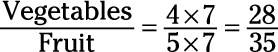Therefore, given that the farmers market has 35 varieties of fruit, it has 28 varieties of vegetables.

2. 63. To begin, set up a proportion based on the ratio of sculptures to paintings: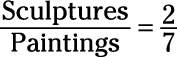Now, increase the terms of the fraction 2/7 so that the number representing sculptures becomes 18: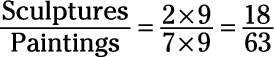Therefore, the gallery has 63 paintings.

3. 208. To begin, set up a proportion based on the ratio of girls to boys: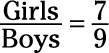Now, you want to increase the terms of the fraction 7/9 so that the number representing boys becomes 117. (To do this, notice that 117 / 9 = 13, so 9 / 13 = 117):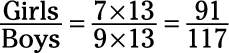Therefore, the camp has 91 girls and 117 boys, so the total number of children is 208.

4. \$825,000. To begin, set up a proportion based on the ratio of state to municipal funding: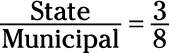Now, you want to increase the terms of the fraction 3/8 so that the number representing municipal funding becomes 600,000. (To do this, notice that 600,000 / 8 = 75,000, so 8 x 75,000 = 600,000):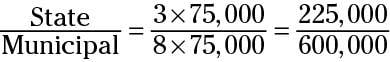Therefore, the town’s budget includes \$225,000 in state funding and \$600,000 in municipal funding, so the total budget is \$825,000.